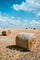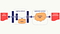# The Fact Registry

## Proofs, DApps, and Economies of Scale

This is a slightly modified version of what we posted on ethresearchcompression in the wild | Photo by Niklas Hamann on Unsplash

# Introduction

In this post we introduce a design pattern for smart-contracts, which we call the Fact Registry Contract pattern, or, simply Registry Contract. This pattern provides a way to separate the verification of a statement from the application that relies on its validity.

The idea is to write a separate contract whose sole purpose is to validate and record elements that satisfy this statement. A statement is defined by a predicate P(x, witness) that gets an element x and some auxiliary information witness and returns either True or False. We will refer to x as a fact, if some witness is known to someone such that P returns True.

Some examples of statements include:

1. isComposite(x, witness) returns True if and only if x is a composite number. The witness is a divisor of x.
2. merkleLeaf((leaf, root), witness) returns True if and only if leaf is a leaf in some Merkle tree with root root. The witness is the relevant authentication path in the tree.
3. signedText((text, public_key), witness) returns True if and only if text appears in some document signed by public_key. The witness is a document containing the text along with its signature.

Checking the above statements may be required as part of some application contract. The common way to deal with such predicates is to write a function inside the application contract and to pass the witness in the calldata of the transaction.

However, in some cases a better approach would be to write a dedicated registry contract. A registry contract is a contract that maintains a set of previously witnessed facts (kept on Ethereum’s storage) and has two methods: add() and isValid(). The add(fact, witness) method adds the fact to the set, provided that the statement holds. The isValid(fact) method simply returns True if the fact is in the set.

In many cases, the size of a fact (excluding the witness) is larger than 32 bytes. In such cases, it is better to maintain the set of hashes of previously witnessed facts, instead of the facts themselves.

Notice that if isValid(fact) returns True, this implies that not only there exists a witness satisfying the predicate, but also that such a witness was presented previously to the contract. As such, the isValid() method serves as a proof of knowledge.Figure 1: The data flow, divided into phases. In phase (1) the fact and the corresponding witness are passed to the registry contract, which verifies validity and adds the fact to the set. In phase (2) when the application contract is invoked, it calls the registry contract to check the validity of the fact.

# When to Use Registry Contracts?

Using a registry contract introduces several overheads compared to the monolithic solution of using a function inside the application contract:

1. One storage operation for every validated fact, resulting from adding the fact to the on-chain set.
2. Transmitting the facts several times: once for the registry contract, and once for every application contract using it.
3. Inter-contract communication while querying the registry contract for fact validity.

When the total cost (computation and calldata) of verifying the statement is low, using registry contracts may add a non-negligible overhead in gas cost and in such a case the monolithic solution is better.

However, when the cost of verification is high, these overheads become negligible and there are many advantages to using the proposed design pattern:

1. Clean separation between the application contract and the registry contract, including the ability to upgrade one without changing the other.
2. Splitting the gas cost over more than one transaction. This is useful when you encounter a situation where the gas cost required for the entire operation is greater than the block limit.
3. Splitting the gas cost between different parties. This is useful when one party should pay for verifying the statement and another party should pay for the application contract referencing the validated fact.
4. Reusing a fact many times (i.e., caching), by different transactions in a single contract, or by different contracts.
5. Economies of Scale: by using a succinct proof system, such as STARK, SNARK or BulletProofs, it is possible to verify a batch of facts using considerably less gas than the total amount of gas required for verifying each fact separately. Thus, different contracts relying on the same statement (not even the same data!) can benefit from massive economies of scale.

Let us elaborate more on this very last benefit, which we view as having tremendous potential.

# Batching with Succinct Proofs of Knowledge

In the examples above, the gas cost of validating facts was additive, in the sense that the gas cost required for validating multiple facts was (approximately) equal to the sum of the costs required for validating the facts separately. However, there are techniques that reduce the amount of gas for validating (or ‘verifying’, in the parlance of proof systems) a batch of facts. For example, succinct proofs of knowledge (like STARKs) allow proving that a certain computation has a given result, with a sublinear cost in the computation size. In particular, proving many facts together is cheaper than the total cost of proving each fact separately.

Consider a registry contract for merkleLeaf() mentioned above. If we extend the function add() to get a batch of new facts (i.e., a list of pairs (leaf, root)), we can check their validity by verifying a succinct proof attesting to the entire batch, instead of checking the authentication path for each and every fact. Note that the authentication paths are not transmitted at all in this case. As the number of facts added to one batch increases, the amortized gas cost per fact decreases (in modern succinct proof systems, the gas cost required for verifying a proof, excluding some small linear cost required for handling the public input, increases (poly-)logarithmically with the batch size).

Succinct proof systems have an efficiency threshold: there is a minimal computational cost associated with verifying a proof, and so it does not make economic sense to generate proofs for batches that fall below a minimum size. Thus, low-bandwidth contracts cannot use such batching mechanisms and gain from their advantages. Registry contracts lower this efficiency threshold by allowing us to gather facts from different applications and place them in one batch. This way, low-bandwidth contracts may benefit from participating in such batches, saving on gas required for fact verification and consequently enabling economies of scale.

Lior Goldberg & Michael Riabzev
StarkWare

Written by

Written by

## StarkWare

#### bringing scalability and privacy to a blockchain near you